# Torque Equation of Three Phase Induction Motor

The torque produced by three phase induction motor depends upon the following three factors:
Firstly the magnitude of rotor current, secondly the flux which interact with the rotor of three phase induction motor and is responsible for producing emf in the rotor part of induction motor, lastly the power factor of rotor of the three phase induction motor.
Combining all these factors, we get the equation of torque as-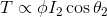Where, T is the torque produced by the induction motor,
φ is flux responsible for producing induced emf,
I2 is rotor current,
cosθ2 is the power factor of rotor circuit.

The flux φ produced by the stator is proportional to stator emf E1.
i.e φ ∝ E1
We know that transformation ratio K is defined as the ratio of secondary voltage (rotor voltage) to that of primary voltage (stator voltage).Rotor current I2 is defined as the ratio of rotor induced emf under running condition , sE2 to total impedance, Z2 of rotor side,and total impedance Z2 on rotor side is given by ,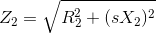Putting this value in above equation we get,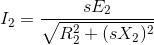s = slip of induction motor

We know that power factor is defined as ratio of resistance to that of impedance. The power factor of the rotor circuit is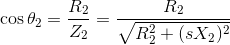Putting the value of flux φ, rotor current I2, power factor cosθ2 in the equation of torque we get,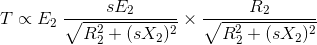Combining similar term we get,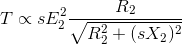Removing proportionality constant we get,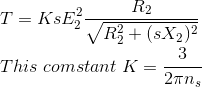Where, ns is synchronous speed in r. p. s, ns = Ns / 60. So, finally the equation of torque becomes,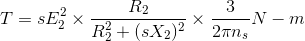Derivation of K in torque equation.
In case of three phase induction motor, there occur copper losses in rotor. These rotor copper losses are expressed as
Pc = 3I22R2
We know that rotor current,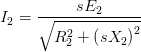Substitute this value of I2 in the equation of rotor copper losses, Pc. So, we getThe ratio of P2 : Pc : Pm = 1 : s : (1 – s)
Where, P2 is the rotor input,
Pc is the rotor copper losses,
Pm is the mechanical power developed.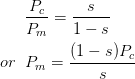Substitute the value of Pc in above equation we get,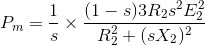On simplifying we get,The mechanical power developed Pm = Tω,Substituting the value of PmWe know that the rotor speed N = Ns(1 – s)
Substituting this value of rotor speed in above equation we get,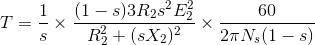Ns is speed in revolution per minute (rpm) and ns is speed in revolution per sec (rps) and the relation between the two is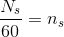Substitute this value of Ns in above equation and simplifying it we getComparing both the equations, we get, constant K = 3 / 2πns

### Equation of Starting Torque of Three Phase Induction Motor

Starting torque is the torque produced by induction motor when it starts. We know that at the start the rotor speed, N is zero.So, the equation of starting torque is easily obtained by simply putting the value of s = 1 in the equation of torque of the three phase induction motor,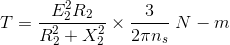The starting torque is also known as standstill torque.

### Maximum Torque Condition for Three-Phase Induction Motor

In the equation of torque,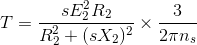The rotor resistance, rotor inductive reactance and synchronous speed of induction motor remain constant. The supply voltage to the three phase induction motor is usually rated and remains constant, so the stator emf also remains the constant. We define the transformation ratio as the ratio of rotor emf to that of stator emf. So if stator emf remains constant, then rotor emf also remains constant.
If we want to find the maximum value of some quantity, then we have to differentiate that quantity concerning some variable parameter and then put it equal to zero. In this case, we have to find the condition for maximum torque, so we have to differentiate torque concerning some variable quantity which is the slip, s in this case as all other parameters in the equation of torque remains constant.
So, for torque to be maximumNow differentiate the above equation by using division rule of differentiation. On differentiating and after putting the terms equal to zero we get,Neglecting the negative value of slip we getSo, when slip s = R2 / X2, the torque will be maximum and this slip is called maximum slip Sm and it is defined as the ratio of rotor resistance to that of rotor reactance.
NOTE: At starting S = 1, so the maximum starting torque occur when rotor resistance is equal to rotor reactance.

### Equation of Maximum Torque

The equation of torque is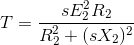The torque will be maximum when slip s = R2 / X2
Substituting the value of this slip in above equation we get the maximum value of torque as,In order to increase the starting torque, extra resistance should be added to the rotor circuit at start and cut out gradually as motor speeds up.
Conclusion
From the above equation it is concluded that

1. The maximum torque is directly proportional to square of rotor induced emf at the standstill.
2. The maximum torque is inversely proportional to rotor reactance.
3. The maximum torque is independent of rotor resistance.
4. The slip at which maximum torque occur depends upon rotor resistance, R2. So, by varying the rotor resistance, maximum torque can be obtained at any required slip.

Want To Learn Faster? 🎓
Get electrical articles delivered to your inbox every week.
No credit card required—it’s 100% free.